# High School Math : How to find the height of an equilateral triangle

## Example Questions

### Example Question #1 : How To Find The Height Of An Equilateral Triangle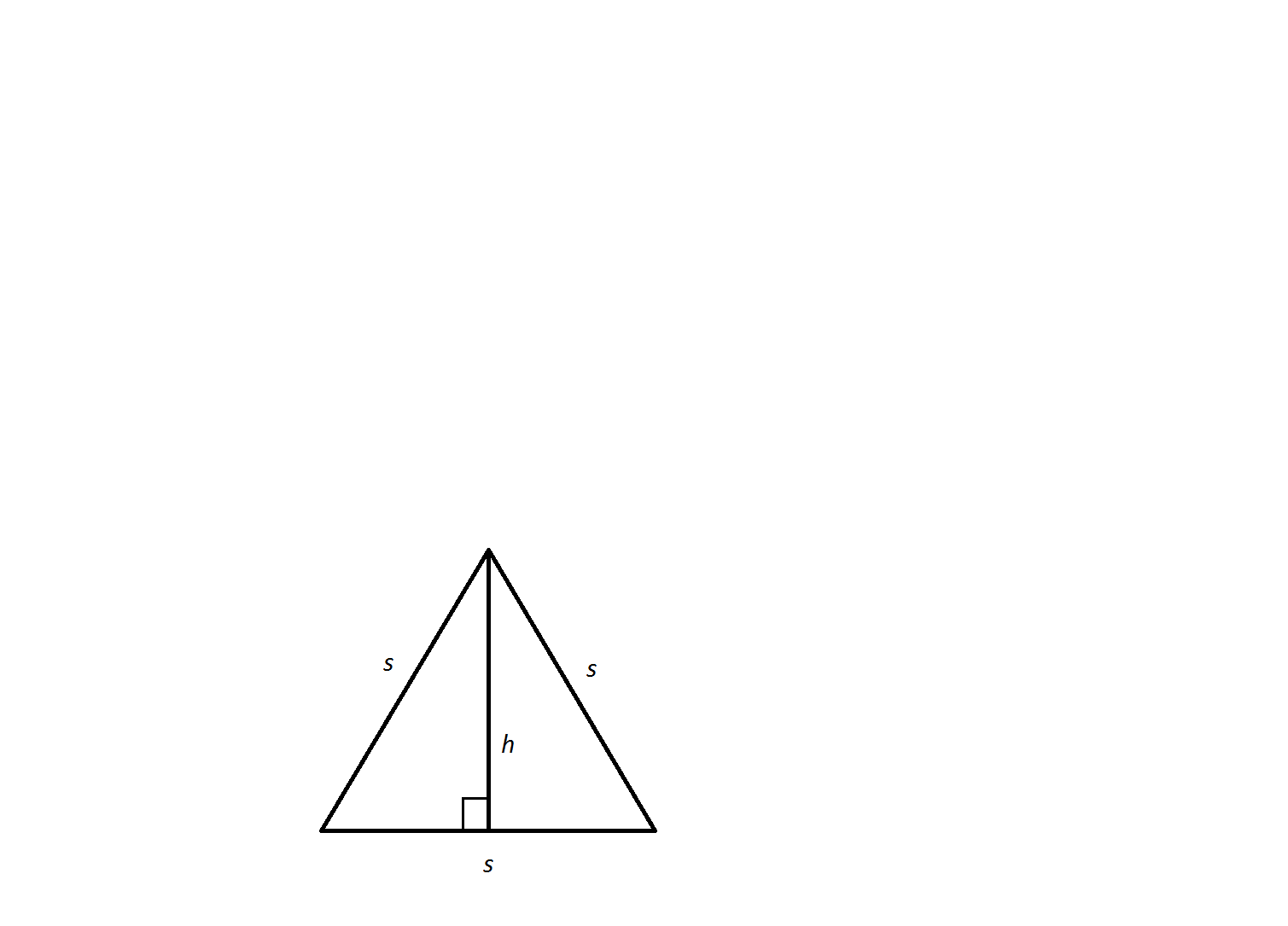An equilateral triangle has a side length of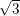. What is the triangle's height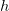?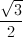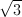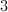Not enough information to solveExplanation:

The altitude,, divides the equilateral triangle into two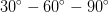right triangles and divides the bottom side in half.

In aright triangle, the sides of the triangle equal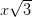, and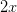. In these equationsequals the length of the smallest side, which in our triangle is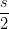or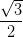.

In this scenario: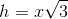and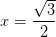Therefore,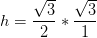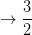### Example Question #2 : How To Find The Height Of An Equilateral TriangleAn equilateral triangle has a side length of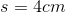.  What is its height,?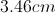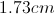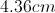Not enough information to solve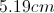Explanation:

An altitude slices an equilateral triangle into two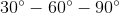triangles. These triangles follow a side-length pattern. The smallest of the two legs equalsand the hypotenuse equals. By way of the Pythagorean Theorem, the longest leg or.

Therefore, we can find the height of the altitude of this triangle by designating a value to. The hypotenuse of one of theis also the side of the original equilateral triangle.  Therefore, one can say that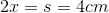and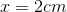.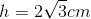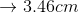### Example Question #3 : How To Find The Height Of An Equilateral Triangle

What is the height of an equilateral triangle with side 6?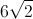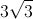Explanation:

When you draw the height in an equilateral triangle, it makes two 30-60-90 triangles. Because of that relationship, the height (which is across from the) is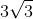### Example Question #4 : How To Find The Height Of An Equilateral Triangle

Find the height of the following equilateral triangle: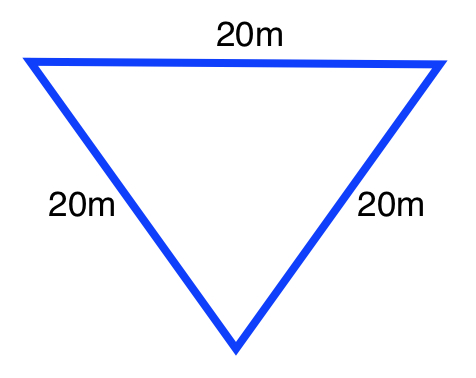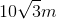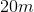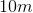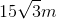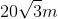Explanation:

Each angle in an equilateral triangle is.

Use the formula for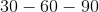triangles in order to find the length of the height.

The formula is: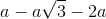Whereis the length of the side opposite the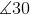If we were to create atriangle by drawing the height, the length of the side is, the base is, and the height is.

### Example Question #5 : How To Find The Height Of An Equilateral Triangle

Solve for the value of X in the following equilateral triangle: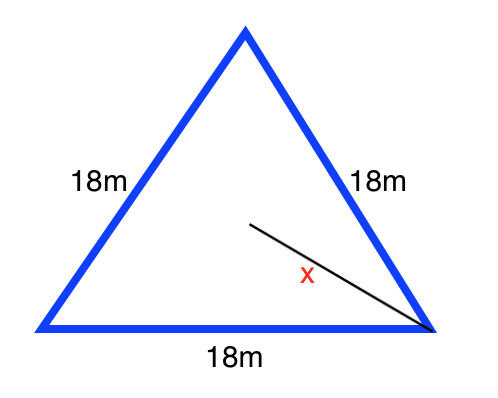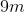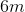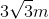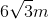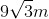Explanation:

If we draw a line segment between X and the base of the triangle, we form atriangle.

We can use the relationships between the sides of atriangle in order to find the length of X.

We know the base opposite the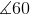is.

The value of the height opposite themust then be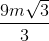, or.

Therefore, the value of X will be twice the value of the height: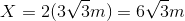### Example Question #3 : How To Find The Height Of An Equilateral Triangle

What is the height of an equilateral triangle with a side length of 8 in?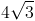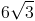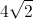Explanation:

An equilateral triangle has three congruent sides, and is also an equiangular triangle with three congruent angles that each meansure 60 degrees.

To find the height we divide the triangle into two special 30 - 60 - 90 right triangles by drawing a line from one corner to the center of the opposite side. This segment will be the height, and will be opposite from one of the 60 degree angles and adjacent to a 30 degree angle. The special right triangle gives side ratios of,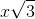, and. The hypoteneuse, the side opposite the 90 degree angle, is the full length of one side of the triangle and is equal to. Using this information, we can find the lengths of each side fo the special triangle.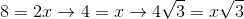The side with lengthwill be the height (opposite the 60 degree angle). The height is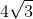inches.